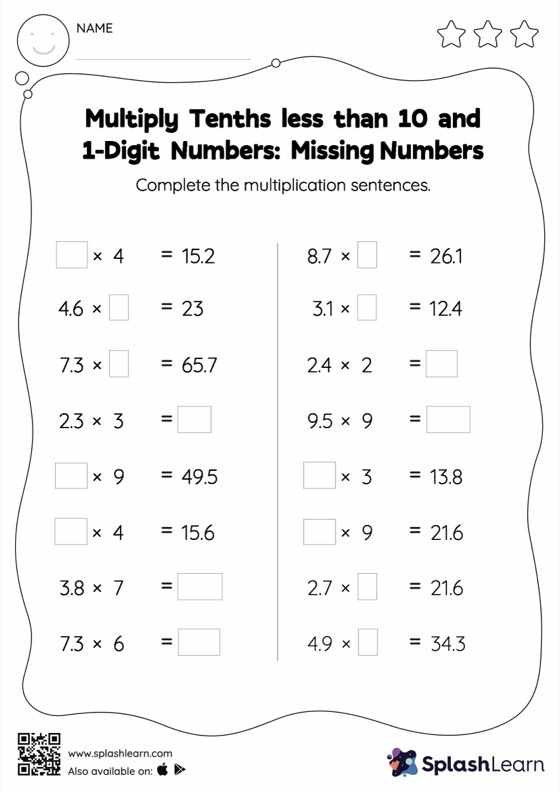# Multiply Tenths less than 10 and 1-Digit Numbers: Missing Numbers Worksheet

Home > Multiply Tenths less than 10 and 1-Digit Numbers: Missing NumbersCan your young mathematician multiply tenths less than 10 and 1-digit numbers? That's exactly what they do here. Students may omit the decimal point when multiplying a decimal by a one-digit number and then add it when writing the answer. Students find missing numbers in multiply tenths less than 10 and 1-digit numbers worksheet using this concept.